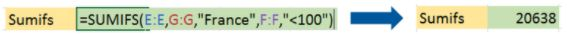Microsoft Excel is the go-to tool for working with data. There are probably a handful of people who haven’t used Excel, given its immense popularity. Excel is a widely used software application in industries today, built to generate reports and business insights. Excel supports several in-built applications that make it easier to use.

One such feature that allows Excel to stand out is – Excel formulas. In this article, we’ll be discussing the various Microsoft Excel functions and formulas. These formulas and functions enable you to perform calculations and data analysis faster. Here, we will look into the top 25 Excel formulas that one must know while working on Excel. The topics that we will be covering  in this article are as follows:

## Excel Formulas and Functions

There are plenty of Excel formulas and functions depending on what kind of operation you want to perform on the dataset. We will look into the formulas and functions on mathematical operations, character-text functions, data and time, sumif-countif, and few lookup functions.

Let’s now look at the top 25 Excel formulas you must know. In this article, we have categorized 25 Excel formulas based on their operations. Let’s start with the first Excel formula on our list.

### 1. SUM

The SUM() function, as the name suggests, gives the total of the selected range of cell values. It performs the mathematical operation which is addition. Here’s an example of it below:

Fig: Sum function in Excel

As you can see above, to find the total amount of sales for every unit, we had to simply type in the function “=SUM(C2:C4)”. This automatically adds up 300, 385, and 480. The result is stored in C5.

### 2. AVERAGE

The AVERAGE() function focuses on calculating the average of the selected range of cell values. As seen from the below example, to find the avg of the total sales, you have to simply type in “AVERAGE(C2, C3, C4)”.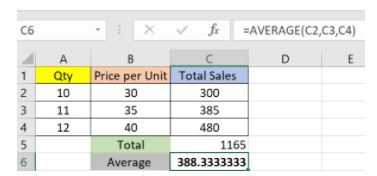Fig: Average function in Excel

It automatically calculates the average, and you can store the result in your desired location.

#### Free Course: Introduction to MS Excel

Master the Fundamentals of MS ExcelEnroll Now### 3. COUNT

The function COUNT() counts the total number of cells in a range that contains a number. It does not include the cell, which is blank, and the ones that hold data in any other format apart from numeric.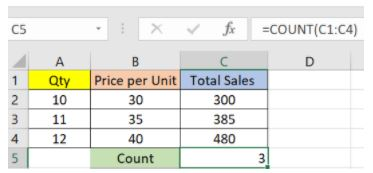Fig: Microsoft Excel Function – Count

As seen above, here, we are counting from C1 to C4, ideally four cells. But since the COUNT function takes only the cells with numerical values into consideration, the answer is 3 as the cell containing “Total Sales” is omitted here.

If you are required to count all the cells with numerical values, text, and any other data format, you must use the function ‘COUNTA()’. However, COUNTA() does not count any blank cells.

To count the number of blank cells present in a range of cells, COUNTBLANK() is used.

### 4. SUBTOTAL

Moving ahead, let’s now understand how the subtotal function works. The SUBTOTAL() function returns the subtotal in a database. Depending on what you want, you can select either average, count, sum, min, max, min, and others. Let’s have a look at two such examples.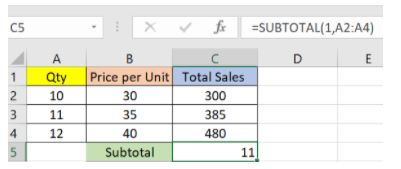Fig: Subtotal function in Excel

In the example above, we have performed the subtotal calculation on cells ranging from A2 to A4. As you can see, the function used is “=SUBTOTAL(1, A2: A4), in the subtotal list “1” refers to average. Hence, the above function will give the average of A2: A4 and the answer to it is 11, which is stored in C5.

Similarly, “=SUBTOTAL(4, A2: A4)” selects the cell with the maximum value from A2 to A4, which is 12. Incorporating “4” in the function provides the maximum result.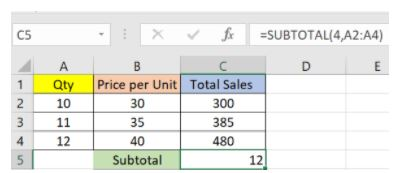Fig: Count function in Excel

### 5. MODULUS

The MOD() function works on returning the remainder when a particular number is divided by a divisor. Let’s now have a look at the examples below for better understanding.

• In the first example, we have divided 10 by 3. The remainder is calculated using the function “=MOD(A2,3)”. The result is stored in B2. We can also directly type “=MOD(10,3)” as it will give the same answer.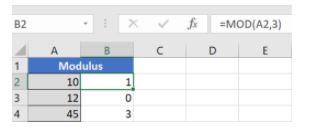Fig: Modulus function in Excel

• Similarly, here, we have divided 12 by 4. The remainder is 0 is, which is stored in B3.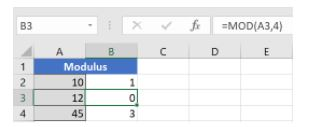Fig: Modulus function in Excel

### 6. POWER

The function “Power()” returns the result of a number raised to a certain power. Let’s have a look at the examples shown below: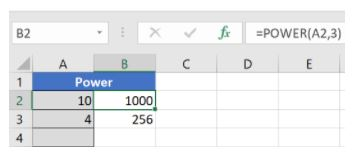Fig: Power function in Excel

As you can see above, to find the power of 10 stored in A2 raised to 3, we have to type “= POWER (A2,3)”. This is how power function works in Excel.

Gain expertise in Business analytics toolsExplore Program### 7. CEILING

Next, we have the ceiling function. The CEILING() function rounds a number up to its nearest multiple of significance.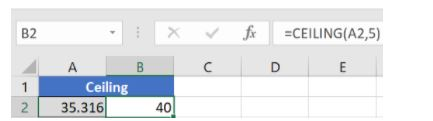Fig: Ceiling function in Excel

The nearest highest multiple of 5 for 35.316 is 40.

### 8. FLOOR

Contrary to the Ceiling function, the floor function rounds a number down to the nearest multiple of significance.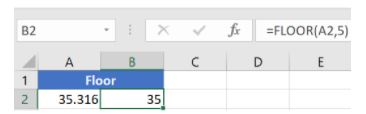Fig: Floor function in Excel

The nearest lowest multiple of 5 for 35.316 is 35.

### 9. CONCATENATE

This function merges or joins several text strings into one text string. Given below are the different ways to perform this function.

• In this example, we have operated with the syntax =CONCATENATE(A25, ” “, B25)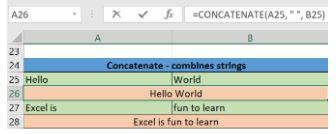Fig: Concatenate function in Excel

• In this example, we have operated with the syntax =CONCATENATE(A27&” “&B27)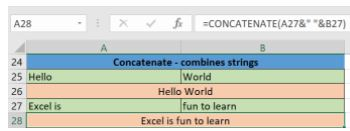Fig: Concatenate function in Excel

Those were the two ways to implement the concatenation operation in Excel.

### 10. LEN

The function LEN() returns the total number of characters in a string. So, it will count the overall characters, including spaces and special characters. Given below is an example of the Len function.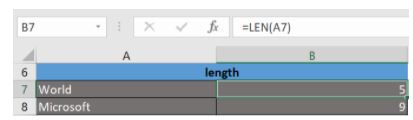Fig: Len function in Excel

Let’s now move onto the next Excel function on our list of this article.

### 11. REPLACE

As the name suggests, the REPLACE() function works on replacing the part of a text string with a different text string.

The syntax is “=REPLACE(old_text, start_num, num_chars, new_text)”. Here, start_num refers to the index position you want to start replacing the characters with. Next, num_chars indicate the number of characters you want to replace.

Let’s have a look at the ways we can use this function.

• Here, we are replacing A101 with B101 by typing “=REPLACE(A15,1,1,”B”)”.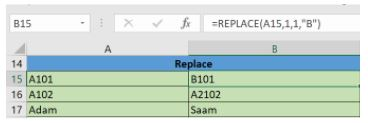Fig: Replace function in Excel

• Next, we are replacing A102 with A2102 by typing “=REPLACE(A16,1,1, “A2”)”.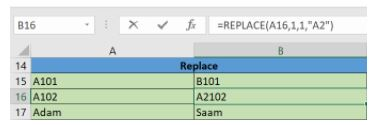Fig: Replace function in Excel

• Finally, we are replacing Adam with Saam by typing “=REPLACE(A17,1,2, “Sa”)”.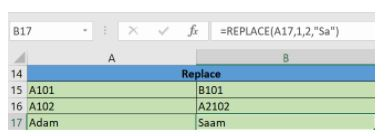Fig: Replace function in Excel

Let’s now move to our next function.

### 12. SUBSTITUTE

The SUBSTITUTE() function replaces the existing text with a new text in a text string.

The syntax is “=SUBSTITUTE(text, old_text, new_text, [instance_num])”.

Here, [instance_num] refers to the index position of the present texts more than once.

Given below are a few examples of this function:

• Here, we are substituting “I like” with “He likes” by typing “=SUBSTITUTE(A20, “I like”,”He likes”)”.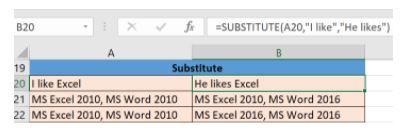Fig: Substitute function in Excel

• Next, we are substituting the second 2010 that occurs in the original text in cell A21 with 2016 by typing “=SUBSTITUTE(A21,2010, 2016,2)”.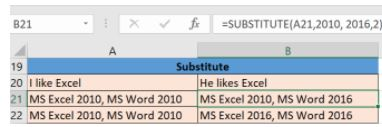Fig: Substitute function in Excel

• Now, we are replacing both the 2010s in the original text with 2016 by typing “=SUBSTITUTE(A22,2010,2016)”.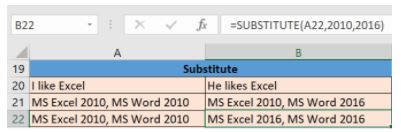Fig: Substitute function in Excel

That was all about the substitute function, let’s now move on to our next function.

#### FREE Business Analytics With Excel Course### 13. LEFT, RIGHT, MID

The LEFT() function gives the number of characters from the start of a text string. Meanwhile, the MID() function returns the characters from the middle of a text string, given a starting position and length. Finally, the right() function returns the number of characters from the end of a text string.

Let’s understand these functions with a few examples.

• In the example below, we use the function left to obtain the leftmost word on the sentence in cell A5.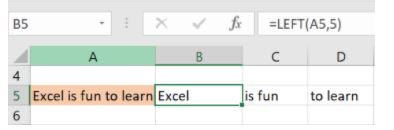Fig: Left function in Excel

Shown below is an example using the mid function.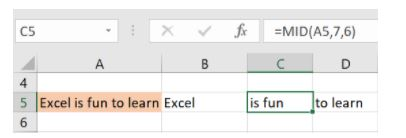Fig: Mid function in Excel

• Here, we have an example of the right function.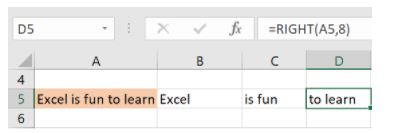Fig: Right function in Excel

### 14. UPPER, LOWER, PROPER

The UPPER() function converts any text string to uppercase. In contrast, the LOWER() function converts any text string to lowercase. The PROPER() function converts any text string to proper case, i.e., the first letter in each word will be in uppercase, and all the other will be in lowercase.

Let’s understand this better with the following examples:

• Here, we have converted the text in A6 to a full uppercase one in A7.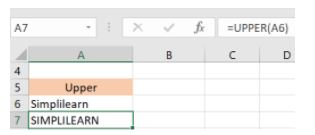Fig: Upper function in Excel

• Now, we have converted the text in A6 to a full lowercase one, as seen in A7.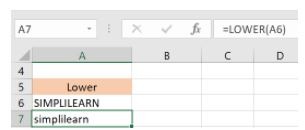Fig: Lower function in Excel

• Finally, we have converted the improper text in A6 to a clean and proper format in A7.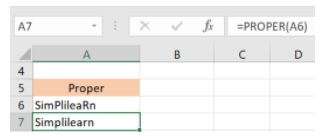Fig: Proper function in Excel

Now, let us hop on to exploring some date and time functions in Excel.

### 15. NOW()

The NOW() function in Excel gives the current system date and time.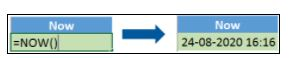Fig: Now function in Excel

The result of the NOW() function will change based on your system date and time.

#### Introduction To Data Analytics (FREE)

Mastery to Data Analytics Basics is a Click Away!Start Learning### 16. TODAY()

The TODAY() function in Excel provides the current system date.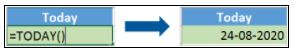Fig: Today function in Excel

The function DAY() is used to return the day of the month. It will be a number between 1 to 31. 1 is the first day of the month, 31 is the last day of the month.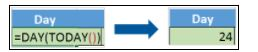Fig: Day function in Excel

The MONTH() function returns the month, a number from 1 to 12, where 1 is January and 12 is December.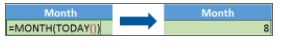Fig: Month function in Excel

The YEAR() function, as the name suggests, returns the year from a date value.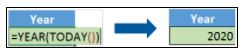Fig: Year function in Excel

### 17. TIME()

The TIME() function converts hours, minutes, seconds given as numbers to an Excel serial number, formatted with a time format.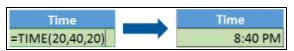Fig: Time function in Excel

### 18. HOUR, MINUTE, SECOND

The HOUR() function generates the hour from a time value as a number from 0 to 23. Here, 0 means 12 AM and 23 is 11 PM.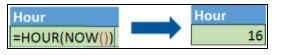Fig: Hour function in Excel

The function MINUTE(), returns the minute from a time value as a number from 0 to 59.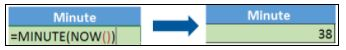Fig: Minute function in Excel

The SECOND() function returns the second from a time value as a number from 0 to 59.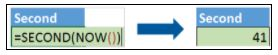Fig: Second function in Excel

### 19. DATEDIF

The DATEDIF() function provides the difference between two dates in terms of years, months, or days.

Below is an example of a DATEDIF function where we calculate the current age of a person based on two given dates, the date of birth and today’s date.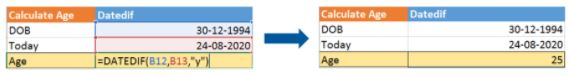Fig: Datedif function in Excel

Now, let’s skin through a few critical advanced functions in Excel that are popularly used to analyze data and create reports.

### 20. VLOOKUP

Next up in this article is the VLOOKUP() function. This stands for the vertical lookup that is responsible for looking for a particular value in the leftmost column of a table. It then returns a value in the same row from a column you specify.

Below are the arguments for the VLOOKUP function:

lookup_value – This is the value that you have to look for in the first column of a table.

table – This indicates the table from which the value is retrieved.

col_index – The column in the table from the value is to be retrieved.

range_lookup – [optional] TRUE = approximate match (default). FALSE = exact match.

We will use the below table to learn how the VLOOKUP function works.

If you wanted to find the department to which Stuart belongs, you could use the VLOOKUP function as shown below: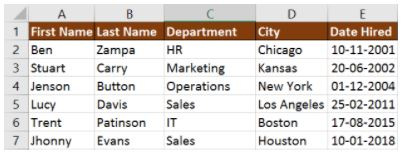Fig: Vlookup function in Excel

Here, A11 cell has the lookup value, A2: E7 is the table array, 3 is the column index number with information about departments, and 0 is the range lookup.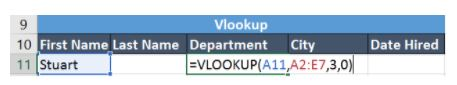If you hit enter, it will return “Marketing”, indicating that Stuart is from the marketing department.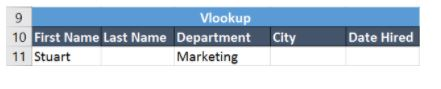### 21. HLOOKUP

Similar to VLOOKUP, we have another function called HLOOKUP() or horizontal lookup. The function HLOOKUP looks for a value in the top row of a table or array of benefits. It gives the value in the same column from a row you specify.

Below are the arguments for the HLOOKUP function:

• lookup_value – This indicates the value to lookup.
• table – This is the table from which you have to retrieve data.
• row_index – This is the row number from which to retrieve data.
• range_lookup – [optional] This is a boolean to indicate an exact match or approximate match. The default value is TRUE, meaning an approximate match.

Given the below table, let’s see how you can find the city of Jenson using HLOOKUP.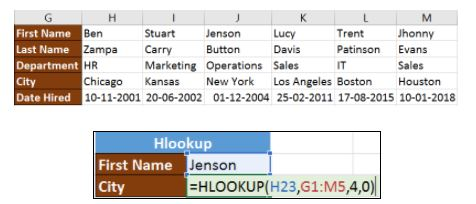Fig: Hlookup function in Excel

Here, H23 has the lookup value, i.e., Jenson, G1:M5 is the table array, 4 is the row index number, 0 is for an approximate match.

Once you hit enter, it will return “New York”.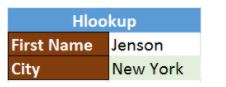### 22. IF

The IF() function checks a given condition and returns a particular value if it is TRUE. It will return another value if the condition is FALSE.

In the below example, we want to check if the value in cell A2 is greater than 5. If it’s greater than 5, the function will return “Yes 4 is greater”, else it will return “No”.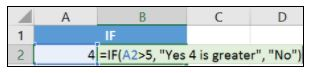Fig: If function in Excel

In this case, it will return ‘No’ since 4 is not greater than 5.

‘IFERROR’ is another function that is popularly used. This function returns a value if an expression evaluates to an error, or else it will return the value of the expression.

Suppose you want to divide 10 by 0. This is an invalid expression, as you can’t divide a number by zero. It will result in an error.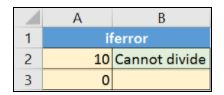The above function will return “Cannot divide”.

### 23. INDEX-MATCH

The INDEX-MATCH function is used to return a value in a column to the left. With VLOOKUP, you’re stuck returning an appraisal from a column to the right. Another reason to use index-match instead of VLOOKUP is that VLOOKUP needs more processing power from Excel. This is because it needs to evaluate the entire table array which you’ve selected. With INDEX-MATCH, Excel only has to consider the lookup column and the return column.

Using the below table, let’s see how you can find the city where Jenson resides.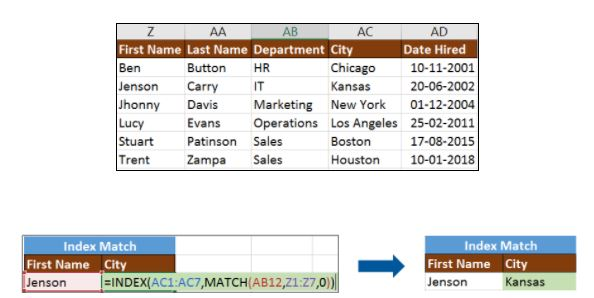Fig: Index-Match function in Excel

Now, let’s find the department of Zampa.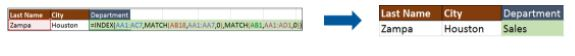### 24. COUNTIF

The function COUNTIF() is used to count the total number of cells within a range that meet the given condition.

Below is a coronavirus sample dataset with information regarding the coronavirus cases and deaths in each country and region.

Let’s find the number of times Afghanistan is present in the table.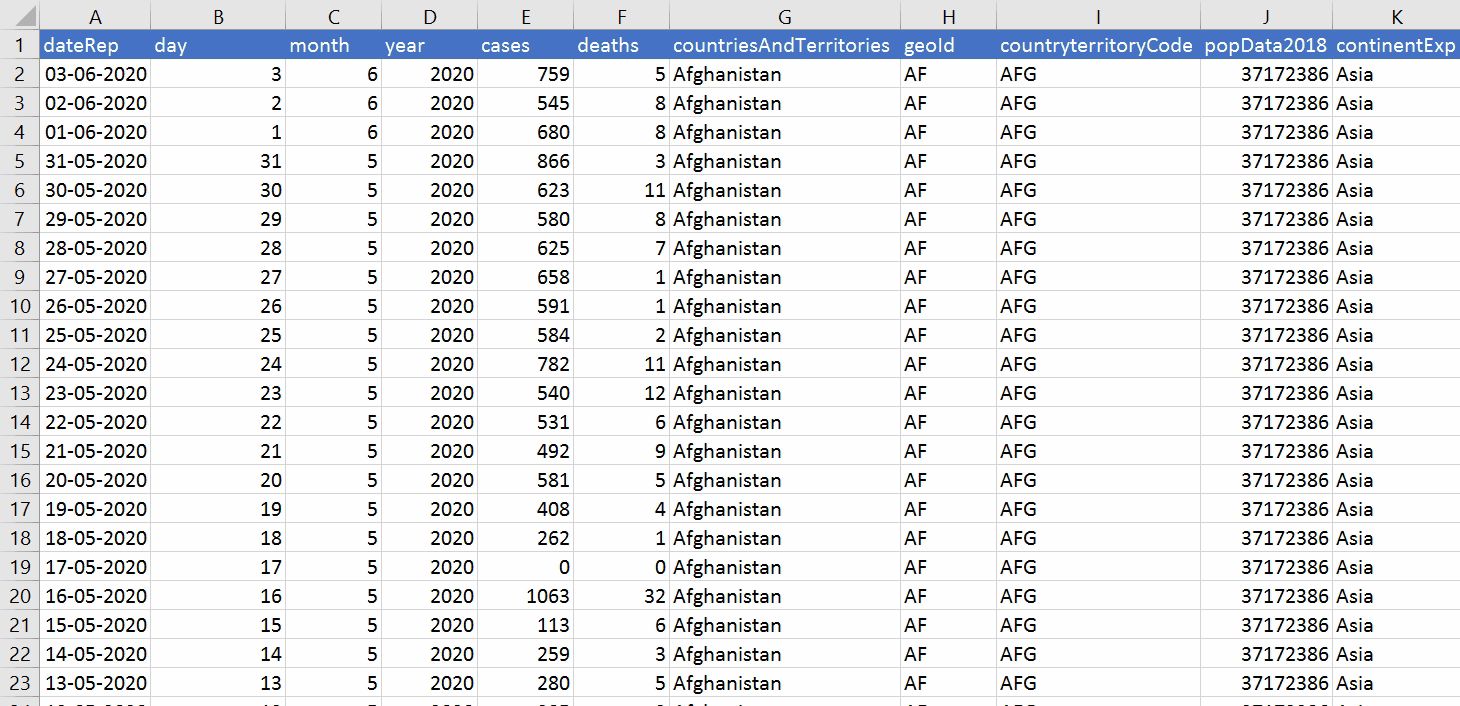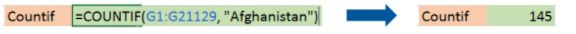Fig: Countif function in Excel

The COUNTIFS function counts the number of cells specified by a given set of conditions.

If you want to count the number of days in which the cases in India have been greater than 100. Here is how you can use the COUNTIFS function.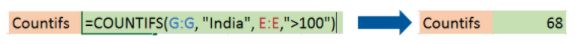### 25. SUMIF

The SUMIF() function adds the cells specified by a given condition or criteria.

Below is the coronavirus dataset using which we will find the total number of cases in India till 3rd Jun 2020. (Our dataset has information from 31st Dec 2020 to 3rd Jun 2020).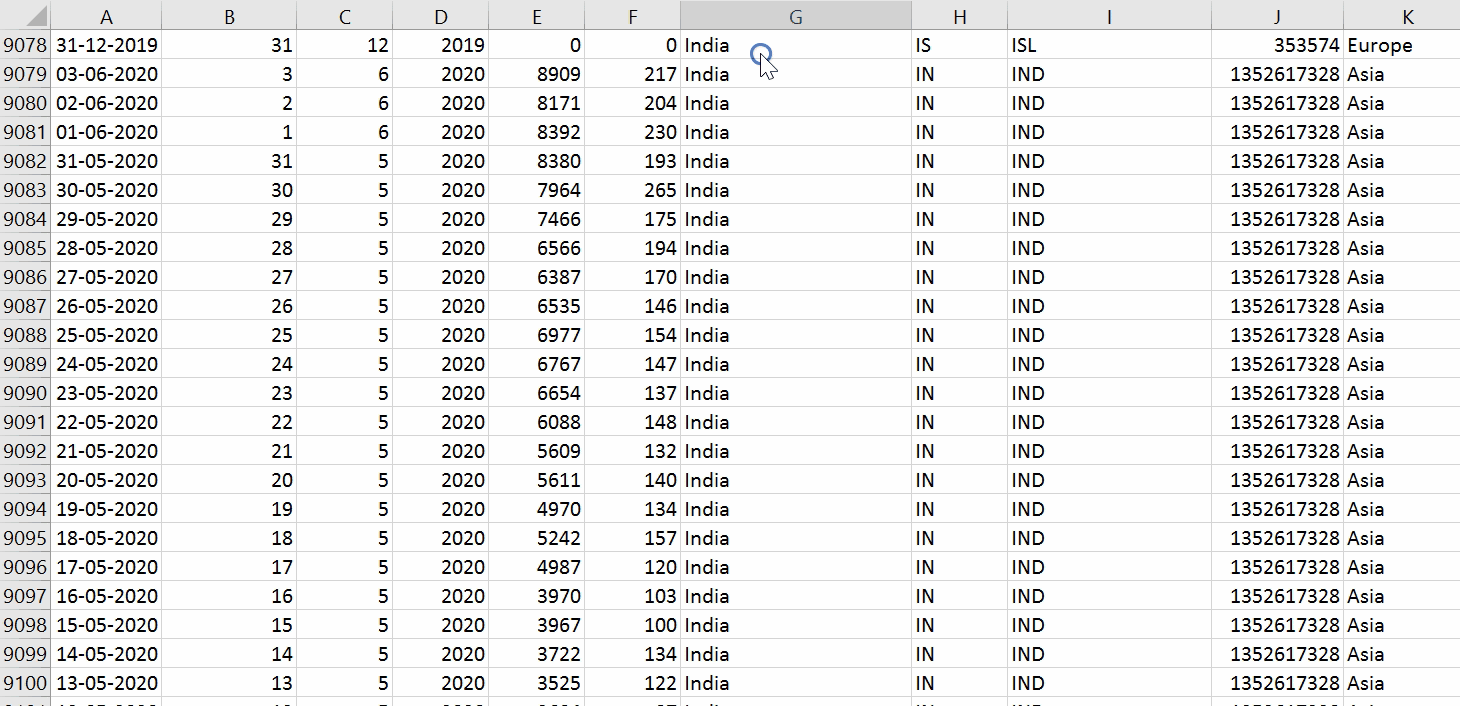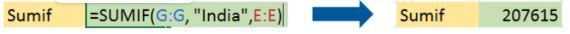Fig: Sumif function in Excel

The SUMIFS() function adds the cells specified by a given set of conditions or criteria.

Let’s find the total cases in France on those days when the deaths have been less than 100.